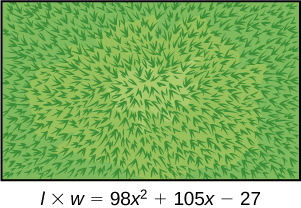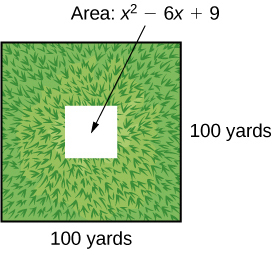# 1.5 Factoring polynomials  (Page 4/6)

 Page 4 / 6

Given a sum of cubes or difference of cubes, factor it.

1. Confirm that the first and last term are cubes, $\text{\hspace{0.17em}}{a}^{3}+{b}^{3}\text{\hspace{0.17em}}$ or $\text{\hspace{0.17em}}{a}^{3}-{b}^{3}.$
2. For a sum of cubes, write the factored form as $\text{\hspace{0.17em}}\left(a+b\right)\left({a}^{2}-ab+{b}^{2}\right).\text{\hspace{0.17em}}$ For a difference of cubes, write the factored form as $\text{\hspace{0.17em}}\left(a-b\right)\left({a}^{2}+ab+{b}^{2}\right).$

## Factoring a sum of cubes

Factor $\text{\hspace{0.17em}}{x}^{3}+512.$

Notice that $\text{\hspace{0.17em}}{x}^{3}\text{\hspace{0.17em}}$ and $\text{\hspace{0.17em}}512\text{\hspace{0.17em}}$ are cubes because $\text{\hspace{0.17em}}{8}^{3}=512.\text{\hspace{0.17em}}$ Rewrite the sum of cubes as $\text{\hspace{0.17em}}\left(x+8\right)\left({x}^{2}-8x+64\right).$

Factor the sum of cubes: $\text{\hspace{0.17em}}216{a}^{3}+{b}^{3}.$

$\left(6a+b\right)\left(36{a}^{2}-6ab+{b}^{2}\right)$

## Factoring a difference of cubes

Factor $\text{\hspace{0.17em}}8{x}^{3}-125.$

Notice that $\text{\hspace{0.17em}}8{x}^{3}\text{\hspace{0.17em}}$ and $\text{\hspace{0.17em}}125\text{\hspace{0.17em}}$ are cubes because $\text{\hspace{0.17em}}8{x}^{3}={\left(2x\right)}^{3}\text{\hspace{0.17em}}$ and $\text{\hspace{0.17em}}125={5}^{3}.\text{\hspace{0.17em}}$ Write the difference of cubes as $\text{\hspace{0.17em}}\left(2x-5\right)\left(4{x}^{2}+10x+25\right).$

Factor the difference of cubes: $\text{\hspace{0.17em}}1,000{x}^{3}-1.$

$\left(10x-1\right)\left(100{x}^{2}+10x+1\right)$

## Factoring expressions with fractional or negative exponents

Expressions with fractional or negative exponents can be factored by pulling out a GCF. Look for the variable or exponent that is common to each term of the expression and pull out that variable or exponent raised to the lowest power. These expressions follow the same factoring rules as those with integer exponents. For instance, $\text{\hspace{0.17em}}2{x}^{\frac{1}{4}}+5{x}^{\frac{3}{4}}\text{\hspace{0.17em}}$ can be factored by pulling out $\text{\hspace{0.17em}}{x}^{\frac{1}{4}}\text{\hspace{0.17em}}$ and being rewritten as $\text{\hspace{0.17em}}{x}^{\frac{1}{4}}\left(2+5{x}^{\frac{1}{2}}\right).$

## Factoring an expression with fractional or negative exponents

Factor $\text{\hspace{0.17em}}3x{\left(x+2\right)}^{\frac{-1}{3}}+4{\left(x+2\right)}^{\frac{2}{3}}.$

Factor out the term with the lowest value of the exponent. In this case, that would be $\text{\hspace{0.17em}}{\left(x+2\right)}^{-\frac{1}{3}}.$

Factor $\text{\hspace{0.17em}}2{\left(5a-1\right)}^{\frac{3}{4}}+7a{\left(5a-1\right)}^{-\frac{1}{4}}.$

${\left(5a-1\right)}^{-\frac{1}{4}}\left(17a-2\right)$

Access these online resources for additional instruction and practice with factoring polynomials.

## Key equations

 difference of squares ${a}^{2}-{b}^{2}=\left(a+b\right)\left(a-b\right)$ perfect square trinomial ${a}^{2}+2ab+{b}^{2}={\left(a+b\right)}^{2}$ sum of cubes ${a}^{3}+{b}^{3}=\left(a+b\right)\left({a}^{2}-ab+{b}^{2}\right)$ difference of cubes ${a}^{3}-{b}^{3}=\left(a-b\right)\left({a}^{2}+ab+{b}^{2}\right)$
• The greatest common factor, or GCF, can be factored out of a polynomial. Checking for a GCF should be the first step in any factoring problem. See [link] .
• Trinomials with leading coefficient 1 can be factored by finding numbers that have a product of the third term and a sum of the second term. See [link] .
• Trinomials can be factored using a process called factoring by grouping. See [link] .
• Perfect square trinomials and the difference of squares are special products and can be factored using equations. See [link] and [link] .
• The sum of cubes and the difference of cubes can be factored using equations. See [link] and [link] .
• Polynomials containing fractional and negative exponents can be factored by pulling out a GCF. See [link] .

## Verbal

If the terms of a polynomial do not have a GCF, does that mean it is not factorable? Explain.

The terms of a polynomial do not have to have a common factor for the entire polynomial to be factorable. For example, $\text{\hspace{0.17em}}4{x}^{2}\text{\hspace{0.17em}}$ and $\text{\hspace{0.17em}}-9{y}^{2}\text{\hspace{0.17em}}$ don’t have a common factor, but the whole polynomial is still factorable: $\text{\hspace{0.17em}}4{x}^{2}-9{y}^{2}=\left(2x+3y\right)\left(2x-3y\right).$

A polynomial is factorable, but it is not a perfect square trinomial or a difference of two squares. Can you factor the polynomial without finding the GCF?

How do you factor by grouping?

Divide the $\text{\hspace{0.17em}}x\text{\hspace{0.17em}}$ term into the sum of two terms, factor each portion of the expression separately, and then factor out the GCF of the entire expression.

## Algebraic

For the following exercises, find the greatest common factor.

$14x+4xy-18x{y}^{2}$

$49m{b}^{2}-35{m}^{2}ba+77m{a}^{2}$

$7m$

$30{x}^{3}y-45{x}^{2}{y}^{2}+135x{y}^{3}$

$200{p}^{3}{m}^{3}-30{p}^{2}{m}^{3}+40{m}^{3}$

$10{m}^{3}$

$36{j}^{4}{k}^{2}-18{j}^{3}{k}^{3}+54{j}^{2}{k}^{4}$

$6{y}^{4}-2{y}^{3}+3{y}^{2}-y$

$y$

For the following exercises, factor by grouping.

$6{x}^{2}+5x-4$

$2{a}^{2}+9a-18$

$\left(2a-3\right)\left(a+6\right)$

$6{c}^{2}+41c+63$

$6{n}^{2}-19n-11$

$\left(3n-11\right)\left(2n+1\right)$

$20{w}^{2}-47w+24$

$2{p}^{2}-5p-7$

$\left(p+1\right)\left(2p-7\right)$

For the following exercises, factor the polynomial.

$7{x}^{2}+48x-7$

$10{h}^{2}-9h-9$

$\left(5h+3\right)\left(2h-3\right)$

$2{b}^{2}-25b-247$

$9{d}^{2}-73d+8$

$\left(9d-1\right)\left(d-8\right)$

$90{v}^{2}-181v+90$

$12{t}^{2}+t-13$

$\left(12t+13\right)\left(t-1\right)$

$2{n}^{2}-n-15$

$16{x}^{2}-100$

$\left(4x+10\right)\left(4x-10\right)$

$25{y}^{2}-196$

$121{p}^{2}-169$

$\left(11p+13\right)\left(11p-13\right)$

$4{m}^{2}-9$

$361{d}^{2}-81$

$\left(19d+9\right)\left(19d-9\right)$

$324{x}^{2}-121$

$144{b}^{2}-25{c}^{2}$

$\left(12b+5c\right)\left(12b-5c\right)$

$16{a}^{2}-8a+1$

$49{n}^{2}+168n+144$

${\left(7n+12\right)}^{2}$

$121{x}^{2}-88x+16$

$225{y}^{2}+120y+16$

${\left(15y+4\right)}^{2}$

${m}^{2}-20m+100$

${m}^{2}-20m+100$

${\left(5p-12\right)}^{2}$

$36{q}^{2}+60q+25$

For the following exercises, factor the polynomials.

${x}^{3}+216$

$\left(x+6\right)\left({x}^{2}-6x+36\right)$

$27{y}^{3}-8$

$125{a}^{3}+343$

$\left(5a+7\right)\left(25{a}^{2}-35a+49\right)$

${b}^{3}-8{d}^{3}$

$64{x}^{3}-125$

$\left(4x-5\right)\left(16{x}^{2}+20x+25\right)$

$729{q}^{3}+1331$

$125{r}^{3}+1,728{s}^{3}$

$\left(5r+12s\right)\left(25{r}^{2}-60rs+144{s}^{2}\right)$

$4x{\left(x-1\right)}^{-\frac{2}{3}}+3{\left(x-1\right)}^{\frac{1}{3}}$

$3c{\left(2c+3\right)}^{-\frac{1}{4}}-5{\left(2c+3\right)}^{\frac{3}{4}}$

${\left(2c+3\right)}^{-\frac{1}{4}}\left(-7c-15\right)$

$3t{\left(10t+3\right)}^{\frac{1}{3}}+7{\left(10t+3\right)}^{\frac{4}{3}}$

$14x{\left(x+2\right)}^{-\frac{2}{5}}+5{\left(x+2\right)}^{\frac{3}{5}}$

${\left(x+2\right)}^{-\frac{2}{5}}\left(19x+10\right)$

$9y{\left(3y-13\right)}^{\frac{1}{5}}-2{\left(3y-13\right)}^{\frac{6}{5}}$

$5z{\left(2z-9\right)}^{-\frac{3}{2}}+11{\left(2z-9\right)}^{-\frac{1}{2}}$

${\left(2z-9\right)}^{-\frac{3}{2}}\left(27z-99\right)$

$6d{\left(2d+3\right)}^{-\frac{1}{6}}+5{\left(2d+3\right)}^{\frac{5}{6}}$

## Real-world applications

For the following exercises, consider this scenario:

Charlotte has appointed a chairperson to lead a city beautification project. The first act is to install statues and fountains in one of the city’s parks. The park is a rectangle with an area of $\text{\hspace{0.17em}}98{x}^{2}+105x-27\text{\hspace{0.17em}}$ m 2 , as shown in the figure below. The length and width of the park are perfect factors of the area.Factor by grouping to find the length and width of the park.

$\left(14x-3\right)\left(7x+9\right)$

A statue is to be placed in the center of the park. The area of the base of the statue is $\text{\hspace{0.17em}}4{x}^{2}+12x+9{\text{m}}^{2}.\text{\hspace{0.17em}}$ Factor the area to find the lengths of the sides of the statue.

At the northwest corner of the park, the city is going to install a fountain. The area of the base of the fountain is $\text{\hspace{0.17em}}9{x}^{2}-25{\text{m}}^{2}.\text{\hspace{0.17em}}$ Factor the area to find the lengths of the sides of the fountain.

$\left(3x+5\right)\left(3x-5\right)$

For the following exercise, consider the following scenario:

A school is installing a flagpole in the central plaza. The plaza is a square with side length 100 yd. as shown in the figure below. The flagpole will take up a square plot with area $\text{\hspace{0.17em}}{x}^{2}-6x+9$ yd 2 .Find the length of the base of the flagpole by factoring.

## Extensions

For the following exercises, factor the polynomials completely.

$16{x}^{4}-200{x}^{2}+625$

${\left(2x+5\right)}^{2}{\left(2x-5\right)}^{2}$

$81{y}^{4}-256$

$16{z}^{4}-2,401{a}^{4}$

$\left(4{z}^{2}+49{a}^{2}\right)\left(2z+7a\right)\left(2z-7a\right)$

$5x{\left(3x+2\right)}^{-\frac{2}{4}}+{\left(12x+8\right)}^{\frac{3}{2}}$

${\left(32{x}^{3}+48{x}^{2}-162x-243\right)}^{-1}$

$\frac{1}{\left(4x+9\right)\left(4x-9\right)\left(2x+3\right)}$

f(x)=x/x+2 given g(x)=1+2x/1-x show that gf(x)=1+2x/3
proof
AUSTINE
sebd me some questions about anything ill solve for yall
how to solve x²=2x+8 factorization?
x=2x+8 x-2x=2x+8-2x x-2x=8 -x=8 -x/-1=8/-1 x=-8 prove: if x=-8 -8=2(-8)+8 -8=-16+8 -8=-8 (PROVEN)
Manifoldee
x=2x+8
Manifoldee
×=2x-8 minus both sides by 2x
Manifoldee
so, x-2x=2x+8-2x
Manifoldee
then cancel out 2x and -2x, cuz 2x-2x is obviously zero
Manifoldee
so it would be like this: x-2x=8
Manifoldee
then we all know that beside the variable is a number (1): (1)x-2x=8
Manifoldee
so we will going to minus that 1-2=-1
Manifoldee
so it would be -x=8
Manifoldee
so next step is to cancel out negative number beside x so we get positive x
Manifoldee
so by doing it you need to divide both side by -1 so it would be like this: (-1x/-1)=(8/-1)
Manifoldee
so -1/-1=1
Manifoldee
so x=-8
Manifoldee
Manifoldee
so we should prove it
Manifoldee
x=2x+8 x-2x=8 -x=8 x=-8 by mantu from India
mantu
lol i just saw its x²
Manifoldee
x²=2x-8 x²-2x=8 -x²=8 x²=-8 square root(x²)=square root(-8) x=sq. root(-8)
Manifoldee
I mean x²=2x+8 by factorization method
Kristof
I think x=-2 or x=4
Kristof
x= 2x+8 ×=8-2x - 2x + x = 8 - x = 8 both sides divided - 1 -×/-1 = 8/-1 × = - 8 //// from somalia
Mohamed
hii
Amit
how are you
Dorbor
well
Biswajit
can u tell me concepts
Gaurav
Find the possible value of 8.5 using moivre's theorem
which of these functions is not uniformly cintinuous on (0, 1)? sinx
which of these functions is not uniformly continuous on 0,1
solve this equation by completing the square 3x-4x-7=0
X=7
Muustapha
=7
mantu
x=7
mantu
3x-4x-7=0 -x=7 x=-7
Kr
x=-7
mantu
9x-16x-49=0 -7x=49 -x=7 x=7
mantu
what's the formula
Modress
-x=7
Modress
new member
siame
what is trigonometry
deals with circles, angles, and triangles. Usually in the form of Soh cah toa or sine, cosine, and tangent
Thomas
solve for me this equational y=2-x
what are you solving for
Alex
solve x
Rubben
you would move everything to the other side leaving x by itself. subtract 2 and divide -1.
Nikki
then I got x=-2
Rubben
it will b -y+2=x
Alex
goodness. I'm sorry. I will let Alex take the wheel.
Nikki
ouky thanks braa
Rubben
I think he drive me safe
Rubben
how to get 8 trigonometric function of tanA=0.5, given SinA=5/13? Can you help me?m
More example of algebra and trigo
What is Indices
If one side only of a triangle is given is it possible to solve for the unkown two sides?
cool
Rubben
kya
Khushnama
please I need help in maths
Okey tell me, what's your problem is?
NavinByByByBy